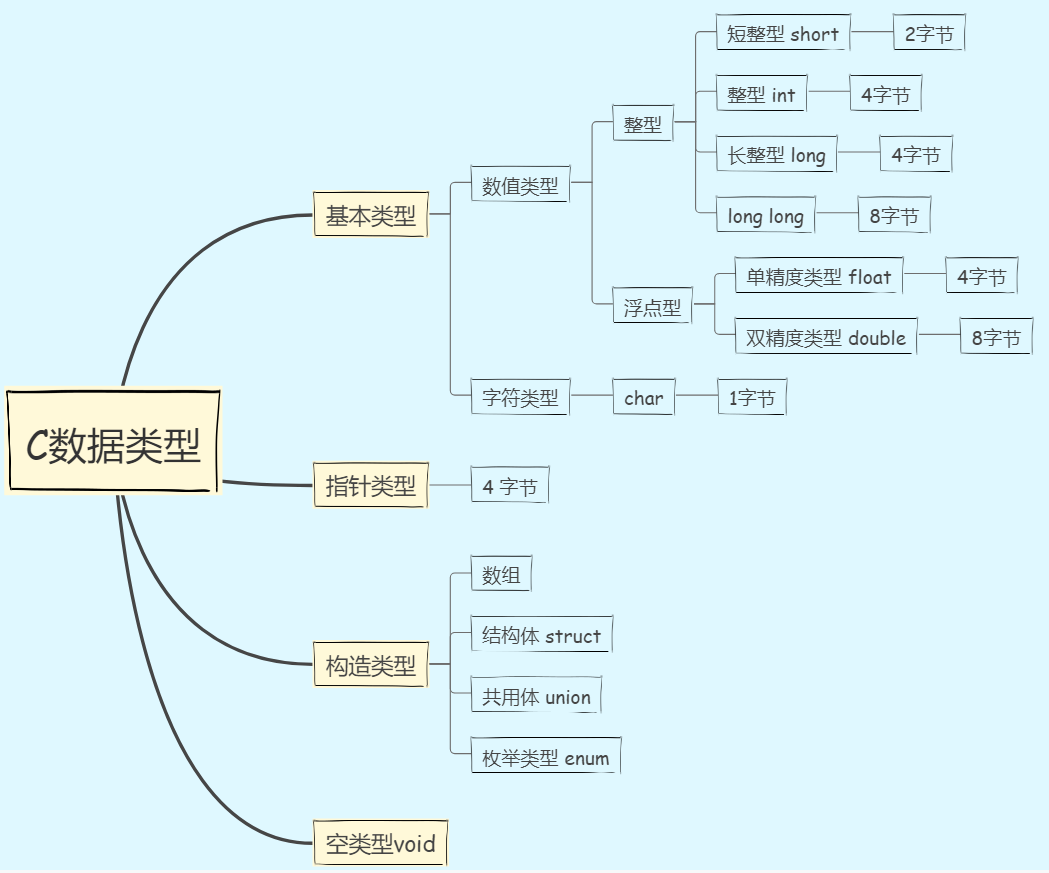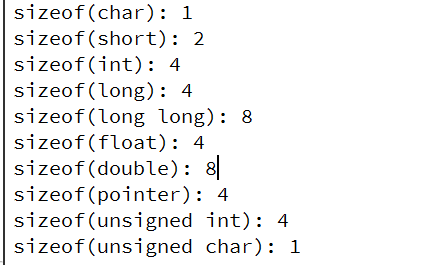# C/C++数据类型和字节

### （1）字节

• 位：位（bit）是计算机存储的最小单位，简记为b,也称为比特(bit),每一位状态只能是0或1。

• 字节：字节（Byte）是计算机用于存储容量的一种计量单位，1个字节可以可以存储1个英文字母或半个汉字。

• 字：是用来一次性处理事务的一个固定长度的位（bit）组。

### （2）位、 字节、 字之间的关系

1字节 (Byte) = 8位（bit）；1字 = 2字节 = 16位；

### （3）计算机的存储

1B(Byte) = 8bit; 1KB = 1024B; 1MB = 1024KB; 1GB = 1024MB; 1TB = 1024GB

### （4）C 数据类型

C数据类型包括基本类型、构造类型、指针类型和空类型void四种。其中基本类型包括数值类型和字符类型(char)；数值类型包括整形和浮点型；整型包括短整型(short)、整型(int)、长整型(long)；浮点型包括单精度型(float)、双精度型(double)；``````cout << "sizeof(char):" << sizeof(char); // 1字节
cout << "sizeof(short):" << sizeof(short); // 2字节
cout << "sizeof(int):" << sizeof(int); // 4字节
cout << "sizeof(long):" << sizeof(long); // 4字节
cout << "sizeof(long long):" << sizeof(long long); // 8字节
cout << "sizeof(float):" << sizeof(float); // 4字节
cout << "sizeof(double):" << sizeof(double);  // 8字节
void* pointer;
cout << "sizeof(pointer):" << sizeof(pointer); // 4字节
cout << "sizeof(unsigned char):" << sizeof(unsigned char); // 1字节
cout << "sizeof(unsigned int):" << sizeof(unsigned int);  // 4字节
``````